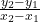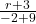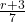## Im trying to do IXL and I dont understand the way my teacher teaches (ironic right?) the points (-9,-3) and (-2,r) fall on a lin

Question

Im trying to do IXL and I dont understand the way my teacher teaches (ironic right?)

the points (-9,-3) and (-2,r) fall on a line with a slope of 1/7. What is the value of r?
If its not right im gonna report you

in progress 0
2 months 2021-07-28T14:35:01+00:00 1 Answers 0 views 0

r = – 2

Step-by-step explanation:

Calculate the slope m using the slope formula and equate tom =with (x₁, y₁ ) = (- 9, – 3) and (x₂, y₂ ) = (- 2, r)

m =, that is=( cross- multiply )

7(r + 3) = 7 ( divide both sides by 7 )

r + 3 = 1 ( subtract 3 from both sides )

r = – 2